Function Repository Resource:

# NetworkBasedInferenceScore

Calculate the network-based inference (NBI) connection score between two vertices in a bipartite graph

Contributed by: Shayan K. Azmoodeh
 ResourceFunction["NetworkBasedInferenceScore"][g,d,t] gives the network based inference (NBI) score between the drug vertex d and the target vertex t in the graph g.

## Details

ResourceFunction["NetworkBasedInferenceScore"] is based on the implementation described by Ding et al. for predicting drug-target interactions in bioinformatics.
ResourceFunction["NetworkBasedInferenceScore"] calculates the score based on how many connections each vertex has and the relation of their connections to each other. It effectively predicts the strength of an edge between two vertices that are not connected by an edge.
The input drug and target vertices do not need to have an edge between them.
If a drug or target vertex given is not in the given graph, ResourceFunction["NetworkBasedInferenceScore"] returns 0.

## Examples

### Basic Examples (2)

Create a simple network of pharmaceutical drugs and their viral targets:

 In:=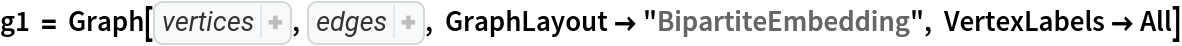Out=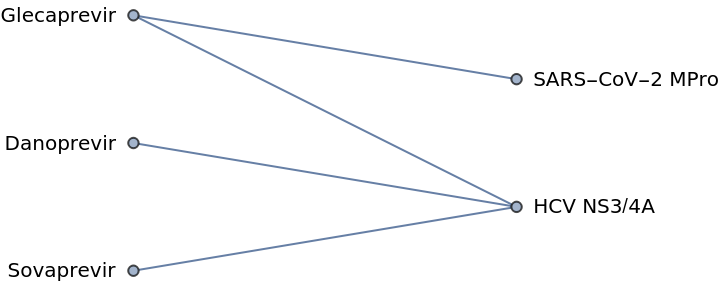Calculate the NBI score between a drug and target vertex:

 In:=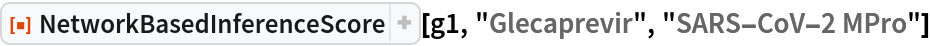Out=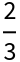A bipartite graph:

 In:=Out=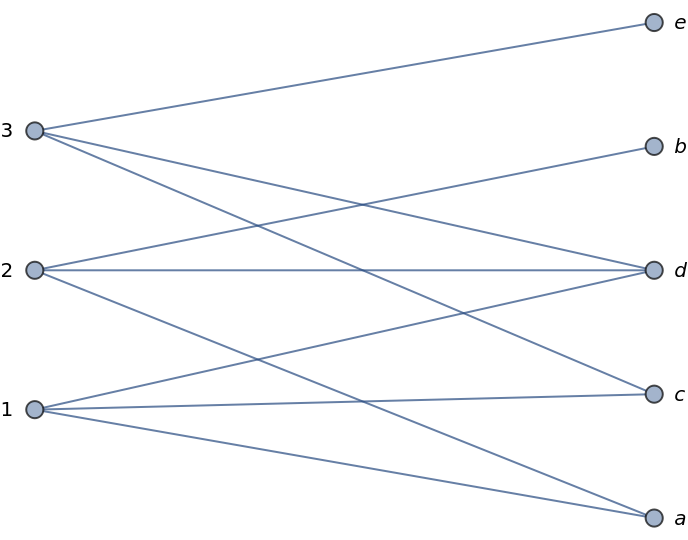The NBI score can be calculated for pairs of vertices that have an edge between them as well:

 In:=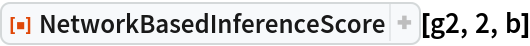Out=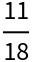### Scope (1)

Network edges can be labeled with the NBI scores:

 In:=In:=Out=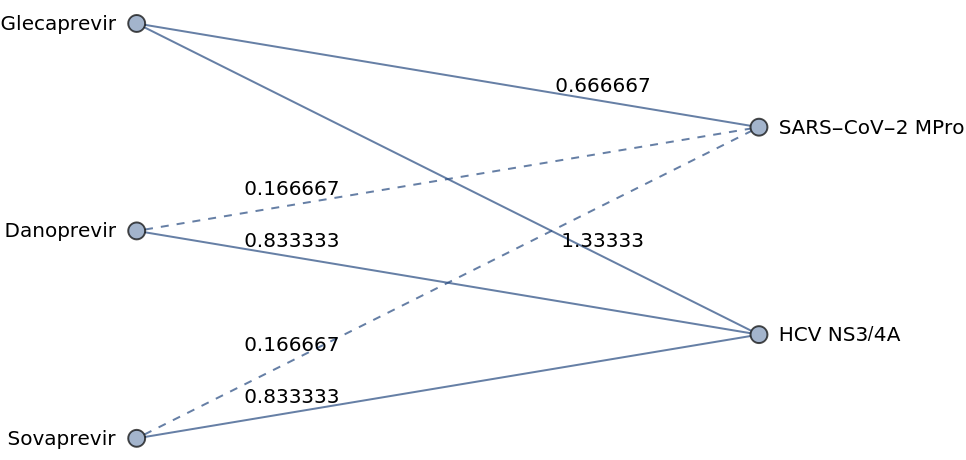Shayan Azmoodeh

## Version History

• 1.0.0 – 14 June 2021